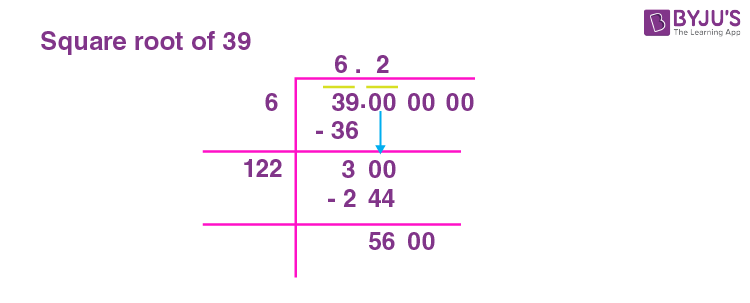# Square Root of 39

The square root of 39 is irrational. The approximate value of the square root of 39 is 7.34. When any number is multiplied by itself, the resultant number is termed the perfect square number and the number that is multiplied is the square root of the obtained product. i.e., If ± i × ± i = p, then i is the square root of p.

The given number 39 lies between 36 and 49, where 36 is obtained by the square of 6 ( ±6 × ±6 = 36) and 49 is obtained by multiplying 7 with 7. (±7 × ±7 = 49).

This means 39 is not a perfect square and does not have a perfect square root. Hence, the square root of 39, √39 is an irrational number. You can refer to Finding Square root with Example for details.

Note the Following:

• The Square root of 39 = √39 = 2√39, where ‘√’ is radical, 39 is the radicand and 2 is the index
• Exponential Form of Square root of 39 = 391/2
• Solution for √39 ≈ 6.2
• The square root of 39 is Irrational = True

## What is the Square root of 39?

The square root of 39 is 6.2 as the square root of 39 is irrational.

 √39 =6.244

## How to Find the Square root of 39?

There are three methods to find the Square root of 39

• Prime Factorisation method
• Long Division method
• Repeated Subtraction method

### Square root of 39 by Prime Factorisation Method

In the prime factorization method, the following steps are followed.

1. Divide 39 with prime factors, starting with 3. The prime factors are 3 × 13.
2. Group the same 2 factors. For 39, there are no 2 same factors. Hence the square root of 39 is √39, which is irrational.

The given number, 39 will be expressed as:

 3 39 13 13 × 1

39 = 3 × 13

Therefore the square root of 39 = √(2 × 13) = √39.

### Square root of 39 by Long Division Method

In the Long Division method, for 39, divide 39 by selecting a divisor such that d × d is less than or equal to 39. If d = 39, then d is the perfect square root of 39, else it is irrational.

Choosing d from the list would be, the squares are:

6 × 6 = 36

7 × 7 = 49Hence 39 is not a perfect square. Therefore 39 is an irrational number.

### Square root of 39 by Repeated Subtraction Method.

In the repeated Subtraction method, 39 is subtracted by odd numbers starting with 1. and the resultant by 3. Next, the result is subtracted by 7 and then by 9 and so on. Check for the difference at any point to become zero. If zero is obtained, then 39 is a perfect square and the step at which it becomes zero is the perfect square root. If the difference does not become zero, then 39 is not a perfect square number and thus won’t have a perfect square root.

The table below shows repeated subtraction for the given number 39.

 Step 1 39 – 1 = 38 Step 2 38 – 3 = 35 Step 3 35 – 5 = 30 Step 4 30 – 7 = 23 Step 5 23 – 9 = 14 Step 6 14 – 11 = 3 Step 7 3 – 13 = -10

Since the difference does not become zero, 39 is not a perfect square number and hence it does not have a perfect square root. The root lies between 7 and 8, resulting in a decimal root.

## Video Lessons

### Visualising square roots### Finding Square roots## Solved Examples

1. Which number is that lesser than or greater than 39, that has a perfect square root?

Solution: 49, which is less than 39 and 64, which is greater than 39, are the perfect square numbers. 7 and 8 are their perfect square roots, respectively.

2. What is the square root of 64?

Solution: 8 is the square root of 64.

## Frequently Asked Questions on Square root of 39

### What is the Square root of 39?

The Square root of 39 is 6.24

### 39 is a perfect square number. True or False?

False. 39 is not a perfect square number.

### What are the prime factors of 39?

The prime factors of 39 are 3 and 13.

### Simplify √39?

√39 = √(3 × 13). No further simplification is possible.

### The square root of 39 is a rational number. True or False?

False. The square root of 39 is not a rational number.